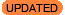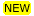# How can I set the axis scaling of plots to linear or logarithmic?Within the Sherpa session, the following commands may be used to set the scale of plots to either linear or logarithmic. Note that the set_* commands allow you to specify that only certain types of plots be affected by the scaling setting, e.g., only data or model plots, as opposed to having all plots created in the session altered.

### Setting the scaling when you create the plotNew in CIAO 4.12 is the ability to change the plot preferences when you make a Sherpa plot. This lets you change one of both of the xlog or ylog settings in the plot_* call. For example:

```sherpa> plot_data(xlog=True)
sherpa> plot_fit(xlog=True, ylog=True)
```

will create a "data" plot with its X axis drawn using a log scale, and then a "fit" plot with both axes drawn with a log scale. Note that the settings applies to both plots for composite plots, so a command like

```sherpa> plot_fit_resid(ylog=True)
```

will use a logarithmic scale for both the fit and residual plots, which is not ideal (since the residual plot should include negative points).

### Changing an existing plot

Matplotlib commands can be used to change existing plots. For example - assuming that import matplotlib.pyplot as plt has already been called - the following will change the Y and then X axes to a logarithmic scale:

```sherpa> plt.yscale('log')
sherpa> plt.xscale('log')
```

If you have a multi-panel plot - e.g. plot_fit_ratio - then you can use the axes attribute of the figure to chose which plot to change. The following example changes the top plot to have a logarithmically-scaled Y axis

```sherpa> fig = plt.gcf()
sherpa> fig = plt.sca(fig.axes)
sherpa> plt.yscale('log')
```

An alternative version would be to use the axis objects directly - as shown below - which uses the set_yscale method of the axis to change the scaling:

```sherpa> ax1, ax2 = fig.axes
sherpa> ax1.set_yscale('log')
```

### Changing the Sherpa preferences

The following commands will change any new plots, but not existing ones.

When called with no arguments, these commands will set the scale of all plots created in the session; otherwise, they will alter only the specified plot type, e.g., 'set_xlog("data")' to set the X axis of only data plots to log scale. They accept the arguments used by the generic plot command, e.g., "data", "model", "source", "fit", "delchi", etc.

The data and model plot preferences can be changed directly (this is similar to using set_xlog and set_ylog).

The get_model_component_plot command must be used to set the axes of plots created with the plot_model_component command.

### Remembering the preference settings

To change the default preference settings for plot_data so that both the X and Y axes of a data plot will be drawn using a log scale each time the function is called in Sherpa, add the following get_data_plot_prefs() functions to your Sherpa customization file, located in \$HOME/.ipython-ciao/profile_sherpa/startup/. For instance, if you create the following file:

```unix% cat > \$HOME/.ipython-ciao/profile_sherpa/startup/99-user.py
print("Setting data/model plots to log scale")
get_data_plot_prefs()["xlog"] = True
get_data_plot_prefs()["ylog"] = True
get_model_plot_prefs()["xlog"] = True
get_model_plot_prefs()["ylog"] = True
```

Then you will see the screen message Setting data/model plots to log scale when you start Sherpa and the plot preferences will be set:

```sherpa> sherpa
-----------------------------------------------------
Welcome to Sherpa: CXC's Modeling and Fitting Package
-----------------------------------------------------
Sherpa 4.12.0

Python 3.7.5 (default, Oct 25 2019, 15:51:11)